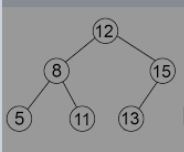New update is available. Click here to update.

# NINJA AND HIS GIRLFRIEND

Last Updated: 28 Mar, 2021
Difficulty: Moderate

## PROBLEM STATEMENT

#### Note:

``````A binary search tree is a binary tree data structure, with the following properties
``````

The left subtree of any node contains nodes with a value less than the node’s value. The right subtree of any node contains nodes with a value equal to or greater than the node’s value. Right, and left subtrees are also binary search trees.

#### Example:

``````consider the following binary search tree, so the minimum absolute difference is between the node value, i.e., | 15-13 |= ‘2’so ‘2’is our answer.
``````#### Input Format:

``````The first line contains an integer ‘T' which denotes the number of test cases or queries to be run. Then the test cases follow.
The first line of each test case contains the tree elements in the level order form separated by a single space.
If any node does not have a left or right child, take -1 in its place. Refer to the example below.

Example:

Elements are in the level order form. The input consists of values of nodes separated by a single space in a single line. In case a node is null, we take -1 in its place.

For example, the input for the tree depicted in the below image would be :
````````````1
2 3
4 -1 5 6
-1 7 -1 -1 -1 -1
-1 -1

Explanation :
Level 1 :
The root node of the tree is 1

Level 2 :
Left child of 1 = 2
Right child of 1 = 3

Level 3 :
Left child of 2 = 4
Right child of 2 = null (-1)
Left child of 3 = 5
Right child of 3 = 6

Level 4 :
Left child of 4 = null (-1)
Right child of 4 = 7
Left child of 5 = null (-1)
Right child of 5 = null (-1)
Left child of 6 = null (-1)
Right child of 6 = null (-1)

Level 5 :
Left child of 7 = null (-1)
Right child of 7 = null (-1)

The first not-null node (of the previous level) is treated as the parent of the first two nodes of the current level. The second not-null node (of the previous level) is treated as the parent node for the next two nodes of the current level and so on.

The input ends when all nodes at the last level are null (-1).
``````
##### Note :
``````The above format was just to provide clarity on how the input is formed for a given tree.

The sequence will be put together in a single line separated by a single space. Hence, for the above-depicted tree, the input will be given as:

1 2 3 -1 5 6 7 -1 -1 -1 -1
``````

#### Output Format:

``````For each test case, print the minimum absolute difference between two different node values.
Print output of each test case in a separate line.
``````

#### Note:

``````You are not required to print anything explicitly. It has already been taken care of. Just implement the function.
``````

#### Constraints:

``````1 <= T <= 5
2 <= N <= 3000
0 <= data <= 10^9

Where ‘T’ is the total number of test cases, ‘N’ is the number of nodes of the tree, and ‘data’ is the value mentioned on the node.

Time Limit: 1 sec
``````## Approach 1

We have to find the minimum difference between two node’s values; suppose we have an array instead of a tree. The minimum difference of two values now we can find by checking each and every pair.

For this, we need a vector for storing all the values; then simply we can find such a pair by iterating our vector with the help of two loops.

## Algorithm:

• Declare an array of integers say, ‘arr.
• ’Then, with the help of queue, we will do the level order traversal of our tree and fill the nodes’ values in our array ‘arr’.
• We use a queue ‘q’ for the level order traversal of our tree and fill our vector ‘arr’ accordingly.
• Now we iterate through our vector by using two nested loops and take the difference of each and every pair and store the minimum in our ‘ans’ variable.
• Now we will simply return ‘ans’.